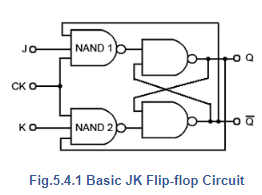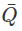Introduction to JK Flip Flops

# Introduction to JK Flip Flops Notes | Study Digital Electronics - Electrical Engineering (EE)

## Document Description: Introduction to JK Flip Flops for Electrical Engineering (EE) 2022 is part of Flip Flops for Digital Electronics preparation. The notes and questions for Introduction to JK Flip Flops have been prepared according to the Electrical Engineering (EE) exam syllabus. Information about Introduction to JK Flip Flops covers topics like and Introduction to JK Flip Flops Example, for Electrical Engineering (EE) 2022 Exam. Find important definitions, questions, notes, meanings, examples, exercises and tests below for Introduction to JK Flip Flops.

Introduction of Introduction to JK Flip Flops in English is available as part of our Digital Electronics for Electrical Engineering (EE) & Introduction to JK Flip Flops in Hindi for Digital Electronics course. Download more important topics related with Flip Flops, notes, lectures and mock test series for Electrical Engineering (EE) Exam by signing up for free. Electrical Engineering (EE): Introduction to JK Flip Flops Notes | Study Digital Electronics - Electrical Engineering (EE)
 1 Crore+ students have signed up on EduRev. Have you?

## JK Flip-flops## A Universal Programmable Flip-flop

The JK Flip-flop is also called a programmable flip-flop because, using its inputs, J, K, S and R, it can be made to mimic the action of any of the other flip-flop types.

Fig. 5.4.1 shows the basic configuration (without S and R inputs) for a JK flip-flop using only four NAND gates. The circuit is similar to the clocked SR flip-flop clocked SR flip-flop shown in Fig. 5.2.7, (Digital Electronics Module 5.2) but in Fig. 5.4.1, it can be seen that although the clock input is the same as in the clocked SR flip-flop, gate NAND 1 in Fig. 5.4.1 is now a three input gate and the set input (S) been replaced by an input labeled J, and the third input provides feedback from the Q output.

On NAND 2 the reset input (R) of Fig 5.2.7 has been replaced by input K and there is an additional feedback connection from Q. The purpose of this feedback is to eliminate the indeterminate state that occurred on the SR flip-flop when both inputs were made logic 0 at the same time.

## Operation

As a starting point, assume that both J and K are at logic 1 and the outputs Q = 0 and= 1, this will cause NAND 1 to be enabled, as it has logic 1 on two (J and) of its three inputs, requiring only a logic 1 on its clock input to change its output state to logic 0. At the same time, NAND 2 is disabled, because it only has one of its inputs (K) at logic 1, its feedback input is at logic 0 because of the feedback from Q.

On the arrival of a clock pulse, the output of NAND 1 therefore becomes logic 0, and causes the flip-flop to change state so that Q = 1 and= 0. This action enables NAND 2 and disables NAND 1.

As this change of state at the outputs occurs however, there is a problem. If the clock pulse is still high, or in its thold period when the flip-flop changes state, the output of NAND 2 will instantly go to logic 0 and the flip-flop will reset back to its original state. This can then set up a situation where the flip-flop will rapidly oscillate between its two states.

These problems caused by the output data ‘racing’ round the feedback lines from output to input before the end of the clock pulse are known as RACE HAZARDS and of course must be avoided. This can be done however, by using a more complex version of the circuit

The document Introduction to JK Flip Flops Notes | Study Digital Electronics - Electrical Engineering (EE) is a part of the Electrical Engineering (EE) Course Digital Electronics.
All you need of Electrical Engineering (EE) at this link: Electrical Engineering (EE)

## Digital Electronics

88 videos|71 docs|8 tests
 Use Code STAYHOME200 and get INR 200 additional OFF

## Digital Electronics

88 videos|71 docs|8 tests

Track your progress, build streaks, highlight & save important lessons and more!

,

,

,

,

,

,

,

,

,

,

,

,

,

,

,

,

,

,

,

,

,

;